AspectRatio

AspectRatio is an option for Show, Plot and other graphic functions.  This discussion is paraphrased from examples provided by Dave Park.  If AspectRatio is set to a number it specifies the height to width ratio of the resulting graphic.  If the AspectRatio is set to Automatic Mathematica sets the width and height so that objects will not be distorted.  Hence the next cell draws a circle so it looks like a circle.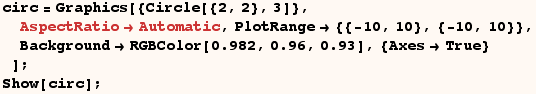In the next cell the AspectRatio is 1/2.  The graphic is not only half as high as it is wide, but the scales along the axes are now also different, and the numbers are packed more closely together in the vertical direction.  Also the circle no longer looks like a circle because of this change of scale.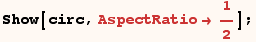In the next cell the graphic is twice as high as it is wide, and the scale is more compressed in the horizontal direction. In fact, some of the numbers overlap, but you can click on the graphic and drag it to a larger size to make it look better.  In this case the circle has been stretched vertically.  Of course the setting need not be written as a rational number.  You could just as well use AspectRatio→2.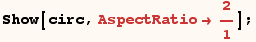The first cell below next cell makes a graphic where the height and width are equal.  This looks very much like the first plot.  However the circle might not look like a circle if a different PlotRange was used as in the second cell below.  The second cell below makes a square graphic, but the circle is stretched because of the PlotRange specified.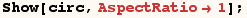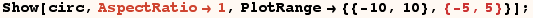Setting AspectRatio to Automatic will always make the scales along the two axes the same and adjusts the length to width ratio as required.  Using Automatic for AspectRatio assures that figures like circles and squares will not be distorted.  On the other hand, setting AspectRatio to a number causes the height to width ratio of the graphic the have the specified value.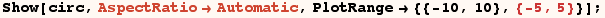If you are making geometrical diagrams you will probably want to set AspectRatio to Automatic. If you are plotting an equation you will more often want to set AspectRatio to a number. If the range of y was 1000 times the range of x then it just wouldn't work to use Automatic. If the plot was one inch wide, it would be 1000 inches high!

In Mathematica the default setting of AspectRatio is usually 1/GoldenRatio. The Golden Ratio was a number determined by the ancient Greeks to give the most pleasing proportion for a rectangle.  It is an interesting mathematical number in itself.  Here is a plot using the Golden Ratio.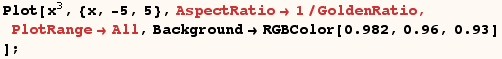It just wouldn't do to use AspectRatio→Automatic for this plot. Try it and see what happens!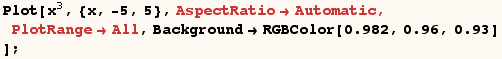One should keep in mind that the ImplicitPlot (from the standard packages) uses  (AspectRatio→Automatic)  by default. The default setting is often preferred since ImplicitPlot is often used to make Plots of circles and related functions.  However, in some situations this default setting will produce a useless graphic.

Created by Mathematica  (May 16, 2004)

Back to Ted’s Tricks index page